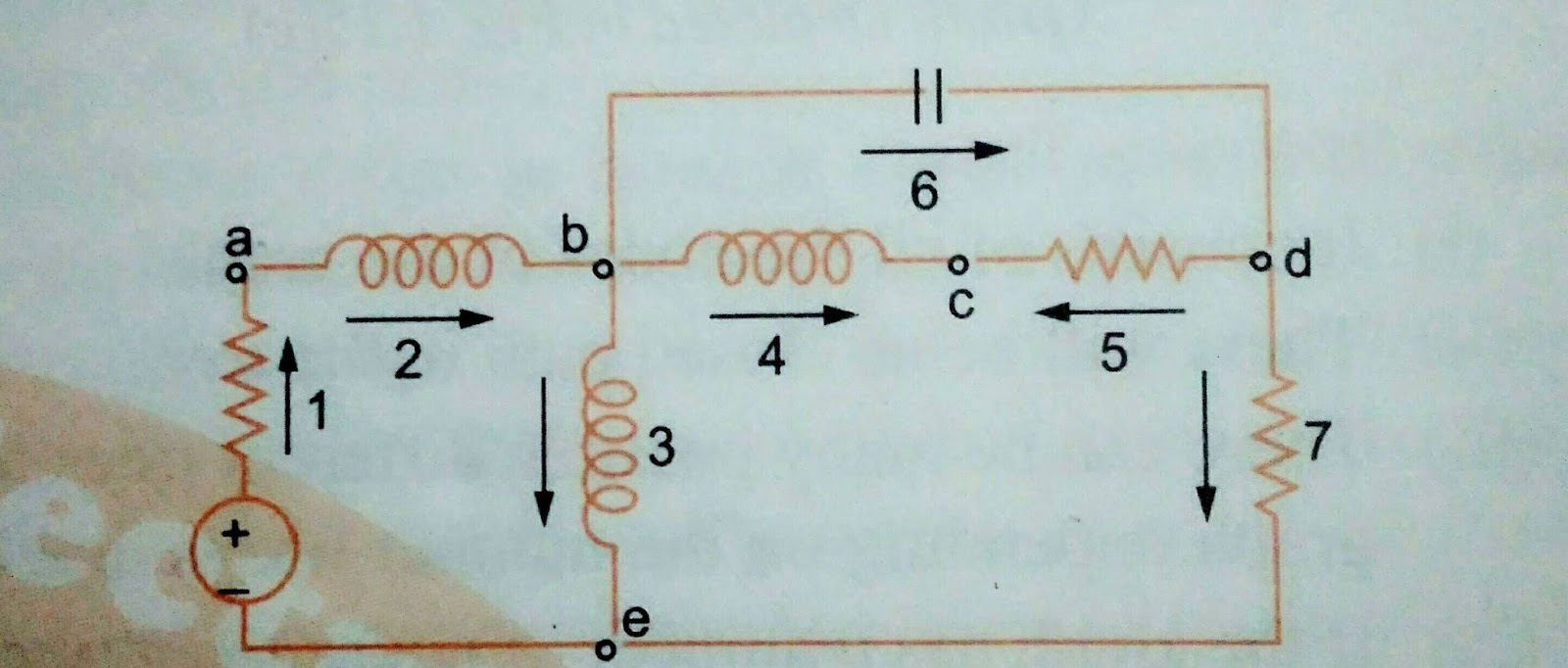### Ticker

6/recent/ticker-posts

# DC BASIC AND NETWORK PART :- 2

ELEMENTS OF GRAPH THEORY

BRANCH AND NODE.

A branch which represents a Circuit element is a line joining two nodes and a nodes is defined as a common point at which two or more Branches meet together.
GRAPH. A liner graph or simply a graph is defined as a collection of node and branches. It's shows the geometrical interconnection of the elements of a network. A graph is connected and of there is a path directly or indirectly between every pairs of nodes. The Circuit of a graph have the following properties.

1) The maximum number of branches in a Circuit will be equal to the number of nodes.

2) There are exactly two paths between any pair of node in a Circuit.
3) There are at least two branches.

A network N and the corresponding graph G in Fig.Networks
(A)Networks
(B)                              (C)

Undirected graph of   Directed ooriented
the Network given in  graph of Network
fig.                                    Given in fig.

Each branch or edge of the graph in fig. Carries and arrow to indicate orientation. A graph whose branches are oriented is called a directed or oriented graph as fig.
Otherwise graph is indirected as fig.

PATH

A path is a improper subgraph such that,
a) At the terminating nodes, only one branch is incident, and
b) At the remaining nodes, two branches are incident.

REQUIREMENTS FOR THE DUAL NETWORKS.

1) Number of mashes in a given Network must be equal to the number of nodes of a dual Network.

2) Total impedance of a given Networks must be equal to the total admittance of a dual Network.

3) Impedance of branch common the two mashes must be equal to the the admittance between two nodes in the equal  Network.

4) Voltage source common to both the loops must be represented as Current source between two nodes.

5) The switch opening at t = 0 in the given Network must be shown as a switch closing at t = 0 in the dual Networks.